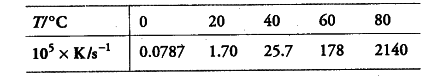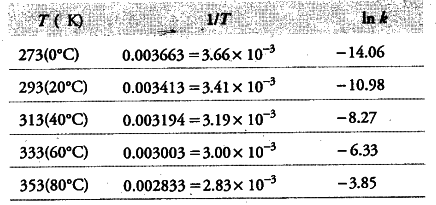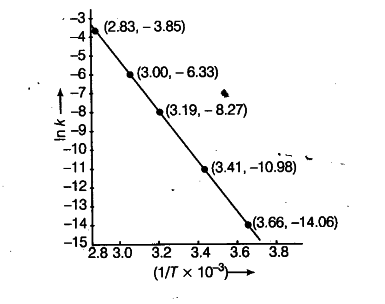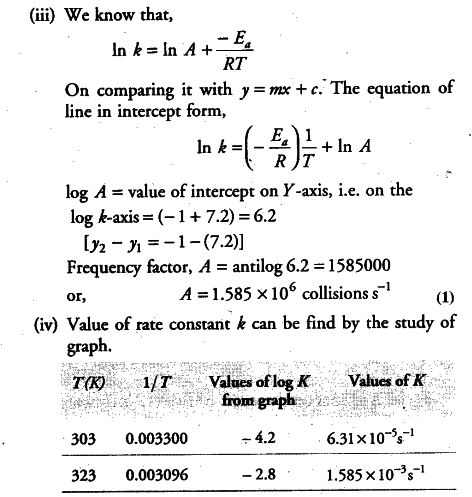# The rate constant for the decomposition of \$N_{2}\$\$O_{5}\$ at various temperatures is given below

The rate constant for the decomposition of \$N_{2}\$\$O_{5}\$ at various temperatures is given below.Draw a graph between In k and 1/T and calculate the values of A . Predict the rate constant at 30°C and 50°C.

(i) To draw the graph between In k versus 1/T, we rewrite the data as follows:(ii) From the graph, we find the slope

Slope = -2.4/0.00047 = -E_{ a }/2.303 R
Activation enery,
E_{ a } = 2.4 x 2.303 x 8.314 J {{mol}^{-1}} /0.00047
= 97772 J {{mol}^{-1}}
= 97.772 kJ {{mol}^{-1}}
===> E_{ a } = 97.772 kJ {{mol}^{-1}}